Kirchhoff's laws / RC Circuits Examples

Example #1

Problem:

Find the currents through all the resistors in the circuit below: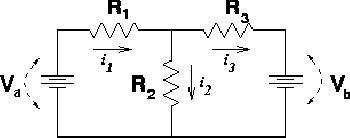DATA: Va = 12 V, Vb = 12 V, R1 = 10 W, R2 = 15 W, R3 = 20 W

Solution:

Summing the voltages around the left and right loops gives the following two equations:

1.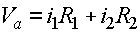2.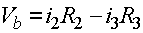3.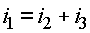One can then reduce the problem to "2 equations 2 unknowns" by substituting for i1 and obtaining 2 new equations.

1.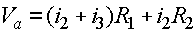2.One can add these two equations together to eliminate the the i3 term since all three resistors are the same, which will be now noted by R.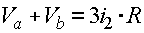Once i2 is known, Eq. (4) can be used to get i3 and i1 can be found by summing i2 + i3.

i2 = 0.554 amps, i1= .369 amps, i3 = -.185 amps

Example #2

Problem:

Find the charges on all the capacitors in the circuit below: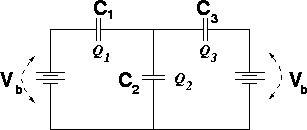DATA: Vb = 12 V, C1 = 10 mF, C2 = 15 mF, C3 = 20 mF

Solution:

Summing the voltages around the left and right loops gives the following two equations

1.2.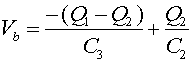where Q3 has been replaced by Q1 - Q2. Dividing Eq. (1) by C3, dividiing Eq. (2) by C1, then adding the equations yields: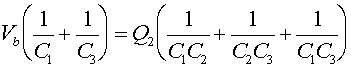which rearranged yields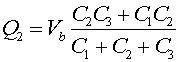Once Q2 is known, Eq. (1) can be used to get Q1, and Q3 can be found as the difference Q1 - Q2.

Q2 = 120.0 mC, Q1= 40.0 mC, Q3 = -80 mC